# Study on the Internal Tension of 7628 fiberglass cloth

7628 fiber7628 fiberglass cloth after weaving the drying process should be carried out at the same time winding packaging, as with the textile process, the process from the empty package to the full package of the packaging process, the 7628 fiber7628 fiberglass cloth must be the tension value of the control.However, the actual tension state in the 7628 fiber7628 fiberglass cloth package is not equal to the tension value controlled at the time of winding, and the process of rolling is decreasing, so that the wrinkles and inter layer slip are easy in the core .In order to solve this problem, the taper taper winding method is more commonly used in the packaging winding, but it is very important to select the appropriate taper coefficient in practical application, especially when the inner radius ratio is large.In this paper, the law of tension change in the process of wrapping and winding is analyzed by elastic mechanics, and the formula of internal tension distribution of taper after tension taper is given. The curvature and taper coefficient are discussed. The final method of determining the taper coefficient for avoiding wrinkles under different package conditions is given.

7628 fiberglass cloth package within the tension calculation

In order to overcome the waste caused by wrinkles at the core of the cloth during the process of wrapping and winding, the size and variation of the internal tension of the cloth roll during the winding process must be analyzed, and the corresponding mathematical model of the packaging winding is established, Finally, by adjusting the winding tension control parameters to avoid or reduce the appearance of wrinkles.

Because of the fact that the tension acting on the fabric in the process of 7628 fiberglass clothis generally the elastic limit, the stress distribution of the cloth roll can be analyzed by the elastic mechanics theory.

In order to facilitate the analysis, we do not imitate the package core radius R0, the entire package to be wrapped in a total of n layer of glass cloth, 7628 fiberglass cloth average thickness of h, then remember Ri = R0 + i * h, (i = 2, …, n) is the outer radius of the package when the i-th layer is wound, whereby Rn is expressed as the maximum outer radius of the package after the package is completed.

We define the three forces associated with the wrapping of the wrapping: one is the rolling tension Ti, (i = 1,2, n), and the cloth is applied to the outer layer, Layer unit width 7628 fiberglass cloth tension,Which is generated by the tension controller; the second is the internal tension Tij, (i

Obviously, there are Tij = δij * h, and the distribution of internal tension and internal stress will change as the winding process progresses, the number of windings and the radius of the reel are increased.In the process of winding the cloth, each new glass 7628 fiber7628 fiberglass cloth will be on the front of the fabric has been rolling inside the pressure, resulting in the internal tension of the internal layers of decline.Since the tension acting on the 7628 fiber7628 fiberglass cloth at the time of winding is within the range of its elastic limit, the elastic distribution principle can be used to analyze the tension distribution in the blanket tube, that is, the inner stress of the i- I + 1 layer, the i + 2 layer, … until the nth layer of rolling tension together results.

In the winding of the outer 7628 fiberglass cloth, mainly through the winding tension on the radial pressure produced by the impact of the internal layers of the fabric stress distribution.See Figure 1, set the current 7628 fiber7628 fiberglass cloth layer, the radius of the cloth tube to Rj, if the surface of the outer 7628 fiber7628 fiberglass cloth on the surface of a pair of uniform radial pressure P, will be in the cloth tube any The i-th layer produces a circumferential stress effect, which is recorded as δ’ij.Figure 1 Effect of internal stress

It is assumed that the core does not undergo deformation during the winding process. For the mechanical problem shown in Fig. 1, the radial pressure P can be obtained by the elasticity principle. The circumferential stress δ’ij caused by the i-th 7628 fiber7628 fiberglass cloth in the cloth tube is: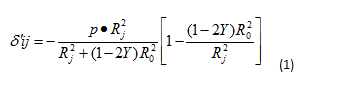Where i<j≤n,Y is the Poisson’s ratio of the fiber fabric.
It is clear that the radial pressure P acting on the outer tube in the process of wrapping the 7628 fiberglass cloth is generated by the roll outer tension Tj, that is, P = Tj / Rj, substituting in equation (1)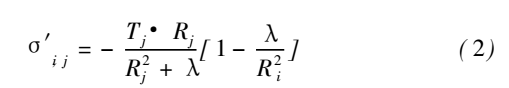Formula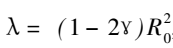With this formula, the effect of the applied tension Tj on the stress distribution in the i-th layer of the cloth roll is obtained when the j-th 7628 fiberglass cloth is wound.
According to the principle of elastic superposition, the internal stress δin acting on the 7628 fiberglass cloth of the i-th layer after the finishing of the n-th 7628 fiberglass cloth can be understood as the influence of the rolling tension Ti and the stress generated by the layers above the i-th layer , I + 1 δi, i + 2 … δi, n and. So you can build the winding 7628 fiberglass cloth tube internal stress distribution formula: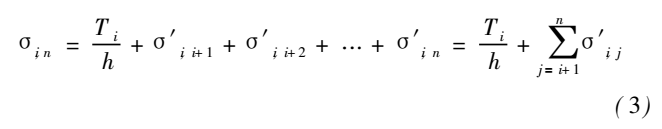Take the formula (2) into can be obtained in the 7628 fiberglass cloth within the layers of fiber in the discrete form of the internal tension distribution: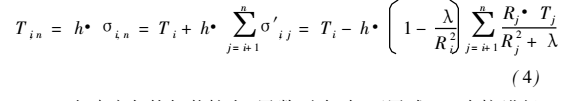When the 7628 fiberglass cloth packaging is small, the number of layers is small, available (4) directly to the cumulative solution.If the package is large, the number of 7628 fiberglass cloth in the package is too high, and the calculation method is still too large by the accumulation method. In this case, it is considered that the average thickness h of the 7628 fiber7628 fiberglass cloth is relatively small, The formula is: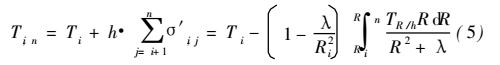The effect of the formula (4) or (5) is obvious, and the tension distribution Tin in the package after the package can be obtained according to the external tension Ti controlled at the time of winding, and it is possible to judge whether the inside of the package is wrinkled (The presence of Tin <0) and the length of the crepe 7628 fiber7628 fiberglass cloth.

7628 fiber7628 fiberglass cloth packaging winding control system
The 7628 fiberglass cloth wrapping control system is composed of tension sensor (TE), photoelectric encoder (PG), tension controller (TC), frequency converter (VVVF), variable frequency motor and take-up roller as shown in Fig.Using the principle of direct tension control, the current coil diameter can be inferred by the photoelectric encoder (PG) and the inverter output, and the tension setting value at this time should be calculated by using the winding tension model in the tension controller. And the tension of the 7628 fiberglass cloth is directly detected by the tension sensor (TE). The output signal is used as the tension feedback amount to produce the tension control closed loop, so that the winding tension can obtain the high performance control effect.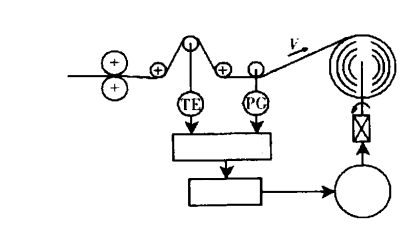Figure 2 Tension control system for 7628 fiberglass cloth packaging process

Tension controller with taper tension winding model, winding volume diameter R, the model control the tension set value: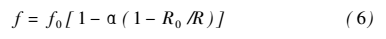In the formula, 0 ≤ α ≤ 1, α is called the taper coefficient, R0 is the core radius, f0 is the initial tension.
At present, the size of 7628 fiberglass cloth has been from the original 300 ~ 500m per volume to each volume of 2000 ~ 4000m, with the package radius increases, the core and the sliding between the layers, the core is not even the uneven wrinkles and other issues.In order to avoid the above problems after packaging, we must analyze the relationship between the relevant control coefficient, package radius and packaging quality, and on this basis to select the taper parameters to ensure the quality of the package.

Internal Tension Analysis and Parameter Determination of Taper Wound Packaging
The taper tension shown in equation (6) can be expressed as follows: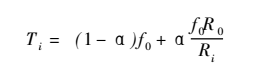In this form, the taper winding tension is composed of two parts, the first part is the constant tension winding part, the second part is the constant torque winding part, the weight is 1-α and α respectively.So the taper coefficient can be adjusted to change the proportion of the two parts, but also by the two parts of the internal tension distribution through the weight of synthetic taper winding tension distribution.

For the constant tension winding, the winding tension Ti = f0, into the formula (5) can be obtained within the constant tension winding tension distribution formula: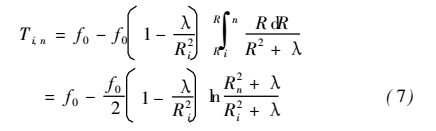From equation (7), if the core radius R0 is given, the tension distribution in the cloth roll is related to the maximum package radius Rn.

For the constant torque winding, the winding tension Ti = (f0R0) / Ri, using the formula (5) can be obtained constant torque winding internal tension distribution formula: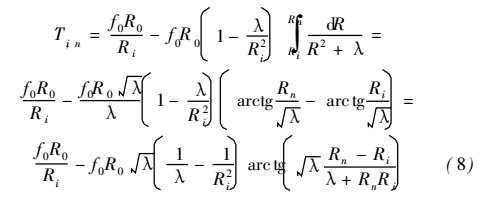Likewise, the tension distribution in the constant torque reel is also related to the maximum radius Rn of the package. Will be constant tension and constant torque winding of the internal tension of the weight of the synthesis can be taper winding tension distribution: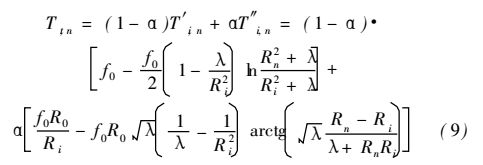Where, T’in, T”in are constant tension, constant torque winding internal tension distribution.
In this way, the internal tension distribution curve of the taper winding is related to the value of the package radius Rn and the taper coefficient α, which can be expressed as Tin (α, Rn). Figure 3 shows the results of the internal tension distribution of the two package radii at different taper coefficients.
It can be seen from the figure that the package radius Rn mainly determines the magnitude of the internal tension, and the taper coefficient α mainly determines the shape of the internal tension distribution curve. The smaller the taper coefficient, the less the internal tension distribution, the greater the internal and external ring tension.
Similarly, in the taper winding, in order to make wrinkles at the core, the inequality min Tin (α, Rn) ≥0 must be established. In this paper, the numerical method is used to calculate the inequality. The relationship between the taper coefficient α and the package radius Rn is not shown in the shaded area.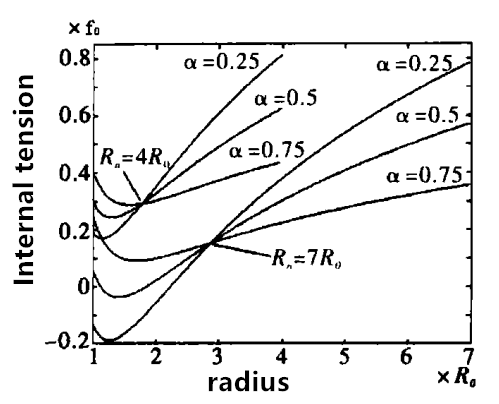Fig.3 Distribution of tension in taper rewinding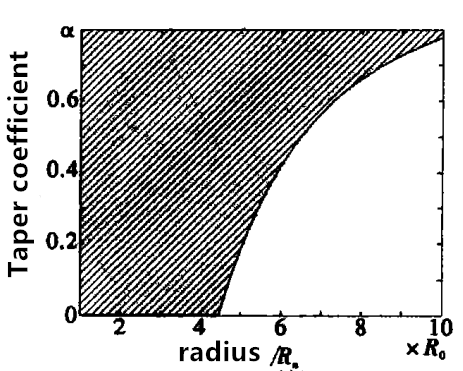Figure 4 No wrinkle condition in taper roll

It can be seen from Figure 4, when the package radius R’n is small, regardless of the taper coefficient to take the value of the winding will not cause wrinkles; when the package radius Rn ≥ 4.45R0, the taper coefficient of the selection of the quality of the impact of winding , The greater the radius of the package, so that after the winding volume does not occur at the wrinkle, taper coefficient selection also increased accordingly.On the other hand, it must be noted that the value of the taper coefficient is not as good as the basic condition that does not occur wrinkles.Too large taper coefficient selection will lead to rapid decline in tension, resulting in outer coil winding tension is too small, loose cloth. Usually can meet the non-wrinkle conditions to leave the remaining 0.1 to select.

Example 1: The packaging of a 7628 fiberglass cloth product, the package control system using taper tension winding mode, the average thickness of 7628 fiberglass cloth = 0.35mm, core radius R0 = 58mm, package size of 1000m, (Rn = 240mm), try the taper coefficient α.
In this example, the maximum package radius is 240mm <4.45R0, so the taper coefficient is chosen to be 0 to 1, and the taper coefficient α = 0.1 is determined in consideration of the selection margin. Example 2: In the above example, if the package size is changed to 2000 m (Rn = 360 mm), the taper coefficient without wrinkling is expected.As the maximum package radius Rn> 4.45R0, so if the coefficient α selected improper, will cause the core wrinkle phenomenon. (9), the inequality minTin (α, Rn) ≥0 is used to determine the selection range of α.The inequality is as follows: When α> 0.47, the inequality constant is established, so the selection range is 0.47 ~ 1, and the taper coefficient α = 0.57 is finally determined after selecting the margin.

Aiming at the taper tension rewinding control system used in the 7628 fiberglass cloth packaging, the tension change and its distribution in the taper of the taper tension,The influence of the radius of the package and the degree of taper on the degree of wrinkling of the cloth roll is discussed. Finally, the method of determining the taper coefficient under different radius of the package is given.Study on the Internal Tension of 7628 fiberglass cloth.pdf